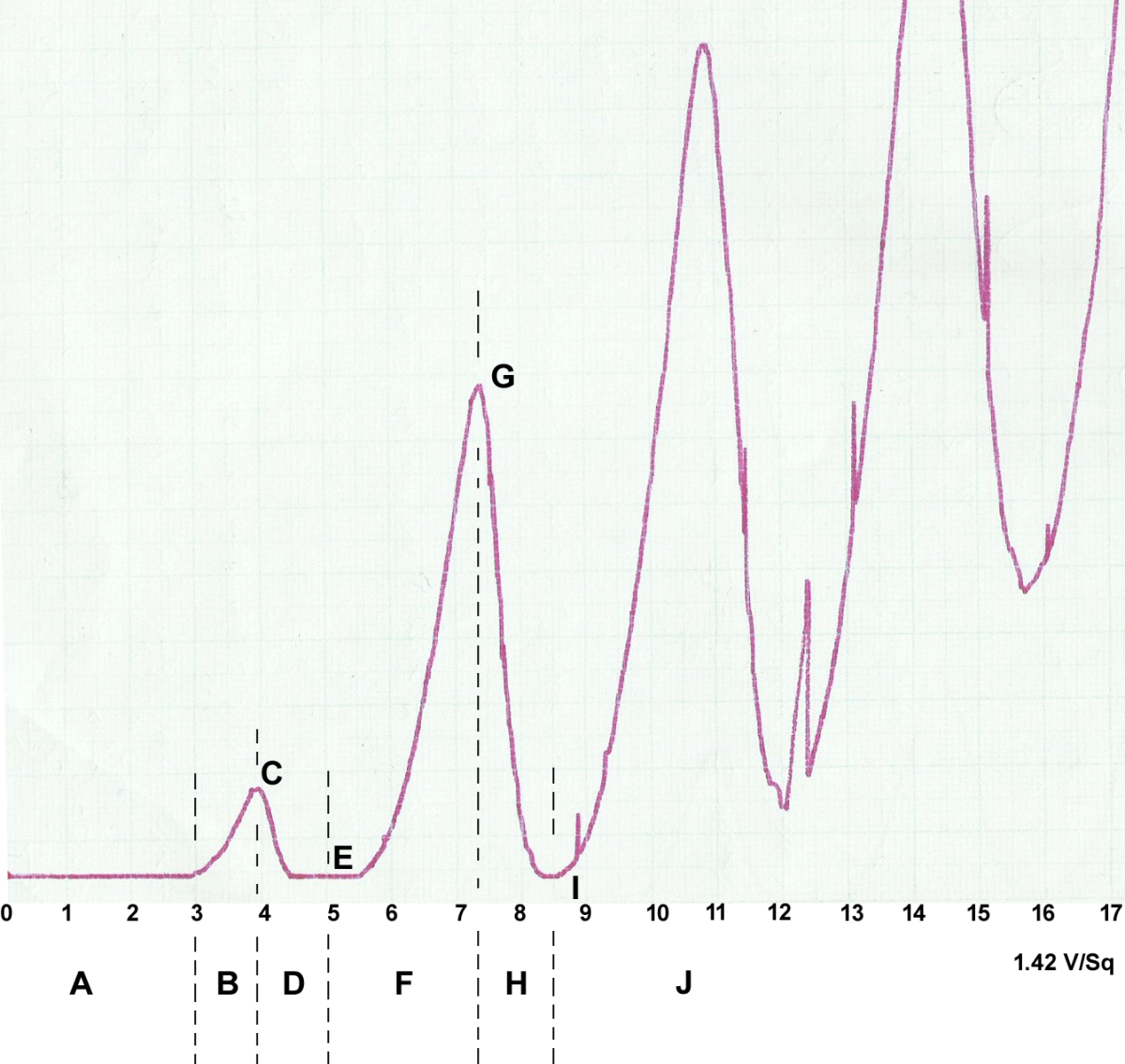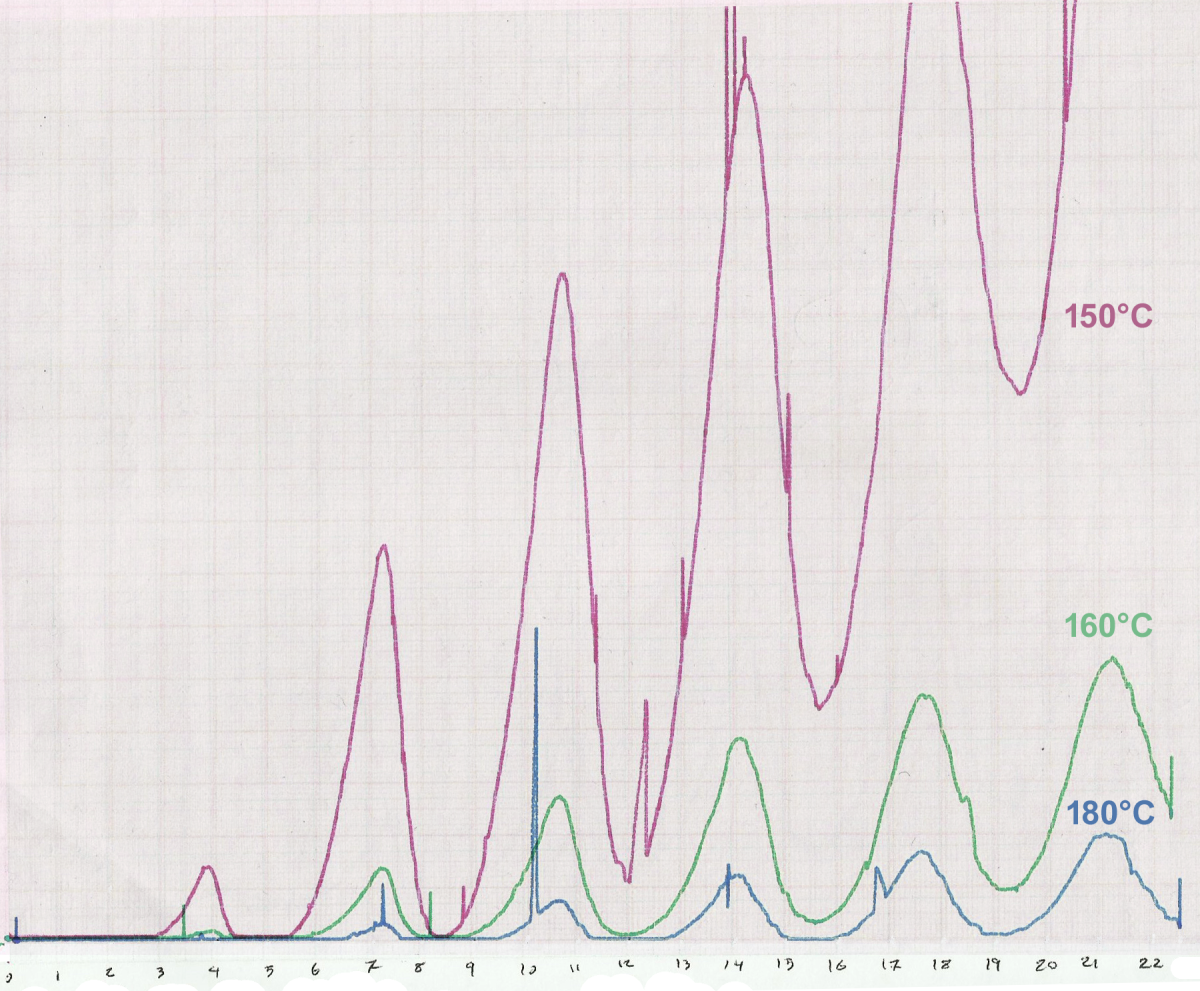# The Franck-Hertz Experiment: A Demonstration of Quantum Theory

Sam Brind holds a master's in physics with theoretical physics (MPhys) from the University of Manchester.

At the start of the 20th century, quantum theory was in its infancy. The basic principle of this new quantum world was that energy was quantised. This means that light can be thought of as being made up of photons, each carrying a unit (or 'quanta') of energy and that electrons occupy discrete energy levels within an atom. These discrete electron energy levels were the key point of the Bohr model of the atom which was introduced in 1913.

The Franck-Hertz experiment, performed by James Franck and Gustav Hertz, was presented in 1914 and clearly demonstrated these discretised energy levels for the first time. It was a historic experiment, acknowledged by the 1925 Nobel Prize in Physics. After a lecture on the experiment, Einstein was reported as saying "It's so lovely, it makes you cry!".

## Experimental Setup

The main part of the experiment is the Franck-Hertz tube which is pictured above. The tube is evacuated to form a vacuum and then filled with an inert gas (typically mercury or neon). The gas is then held at a low pressure and a constant temperature. Typical experiments will involve a temperature control system to allow the tube's temperature to be adjusted. During the experiment the current, I, is measured and will usually be output through an oscilloscope or a graph plotting machine.

Four different voltages are applied across different sections of the tube. We will describe the sections from left to right to fully understand the tube and how a current is produced. The first voltage, UH, is used to heat a metal filament, K. This produces free electrons via thermionic emission (heat energy overcoming the electrons work function to break the electron free from its atom).

Near to the filament is a metal grid, G1, which is held at a voltage, V1. This voltage is used to attract the newly free electrons, which then pass through the grid. An accelerating voltage, U2, is then applied. This accelerates the electrons towards the second grid, G2. This second grid is held at a stopping voltage, U3, which acts to oppose the electrons reaching the collecting anode, A. The electrons collected at this anode produce the measured current. Once the values of UH, U1 and U3 are set the experiment boils down to varying the accelerating voltage and observing the effect on the current.Data collected using mercury vapour heated to 150 Celsius within the Franck-Hertz tube. Current is plotted as a function of accelerating voltage. Note that the general pattern is important and not the sharp jumps which are simply experimental noise.

## Results

Shown in the diagram above is an example of the shape of a typical Franck-Hertz curve. The diagram has been labelled to indicate the key parts. How are the features of the curve accounted for? Assuming the atom has discretised energy levels, there are two types of collision the electrons can have with the gas atoms in the tube:

• Elastic collisions - The electron "bounces" off the gas atom without losing any energy/speed. Only the direction of travel is changed.
• Inelastic collisions - The electron excites the gas atom and loses energy. Due to the discrete energy levels, this can only happen for a precise value of energy. This is called the excitation energy and corresponds to the difference in energy between the atomic ground state (lowest possible energy) and a higher energy level.

A - No current is observed.

The accelerating voltage isn't strong enough to overcome the stopping voltage. Hence, no electrons reach the anode and no current is produced.

B - The current rises to a 1st maximum.

The accelerating voltage becomes sufficient to give the electrons enough energy to overcome the stopping voltage but not enough to excite the gas atoms. As the acceleration voltage increases the electrons have more kinetic energy. This reduces the time to cross the tube and therefore the current increases (I = Q/t).

C - The current is at the 1st maximum.

The accelerating voltage is now sufficient to give electrons enough energy to excite the gas atoms. Inelastic collisions can begin. After an inelastic collision, the electron may not have enough energy to overcome the stopping potential so the current will start to drop.

Scroll to Continue

D - The current drops from the 1st maximum.

Not all electrons are moving at the same speed or even direction, due to elastic collisions with the gas atoms which have their own random thermal motion. Therefore, some electrons will need more accelerating than others to reach the excitation energy. This is why the current gradually drops instead of falling sharply.

E - The current is at the 1st minimum.

A maximum number of collisions exciting the gas atoms is reached. Therefore, a maximum number of electrons are not reaching the anode and there is a minimum current.

F - The current rises again, up to a 2nd maximum.

The accelerating voltage is increased enough to accelerate electrons sufficiently to overcome the stopping potential after they have lost energy to an inelastic collision. The average position of inelastic collisions move leftwards down the tube, closer to the filament. The current rises due to the kinetic energy argument described in B.

G - The current is at the 2nd maximum.

The accelerating voltage is now sufficient to give electrons enough energy to excite 2 gas atoms while it travels the length of the tube. The electron is accelerated, has an inelastic collision, accelerated again, has another inelastic collision and then doesn't have enough energy to overcome the stopping potential so current starts to drop.

H - The current again drops, from the 2nd maximum.

The current drops gradually due to the effect described in D.

I - The current is at the 2nd minimum.

A maximum number of electrons having 2 inelastic collisions with the gas atoms is reached. Therefore, a maximum number of electrons are not reaching the anode and a second minimum current is reached.

J - This pattern of maxima and minima then repeats for higher and higher accelerating voltages.

The pattern then repeats as more and more inelastic collisions are fitted into the length of the tube.

It can be seen that the minima of the Franck-Hertz curves are equally spaced (barring experimental uncertainties). This spacing of the minima is equal to the excitation energy of the gas atoms (for mercury this is 4.9 eV). The observed pattern of equally spaced minima is evidence that the atomic energy levels must be discrete.

## What about the effect of changing the temperature of the tube?

An increase in tube temperature would lead to an increase in the random thermal motion of the gas atoms within the tube. This increases the likelihood of the electrons having more elastic collisions and taking a longer path to the anode. A longer path delays the time to reach the anode. Therefore, increasing temperature increases the average time for the electrons to cross the tube and decreases the current. The current drops as temperature increases and the amplitude of the Franck-Hertz curves will drop but the distinct pattern will remain.Overlaid Franck-Hertz curves for varying temperatures of mercury (demonstrating the expected reduction in amplitude).

Question: What is the purpose of the retarding potential?

Answer: The retarding potential (or 'stopping voltage') prevents low energy electrons from reaching the collecting anode and contributing to the measured current. This greatly enhances the contrast between minima and maxima in current, allowing the distinct pattern to be observed and measured accurately.

M.Behzad afzal on October 13, 2019:

atoms do not ionized because during inelastic collision just their internal energy increases and electron jump into excited state do not leave the atom.

Sam Brind (author) on September 08, 2018:

Ionisation is another possible inelastic collision that the electrons could be involved in. However, it is less likely than an excitation because it requires a higher energy (for Mercury around 10 eV).

At large enough voltages (depending on the gas density, current and tube properties) ionisation will actually cause a discharge. A discharge is when the free electron produced by an ionisation will go on to cause further ionisation. This turns the gas into a conducting plasma and prevents any further measurements for this experiment.

Farhan tariq on September 04, 2018:

Why during inelastic colision atoms becomes exited and do not ionized in frank hertZ exp?

jeff blight on June 13, 2017:

yes mate